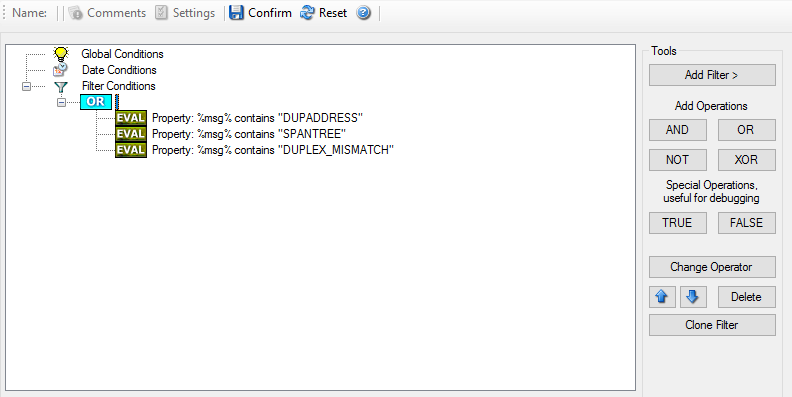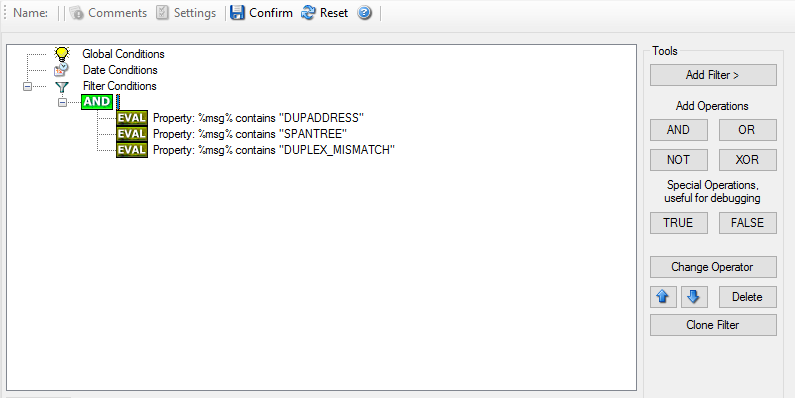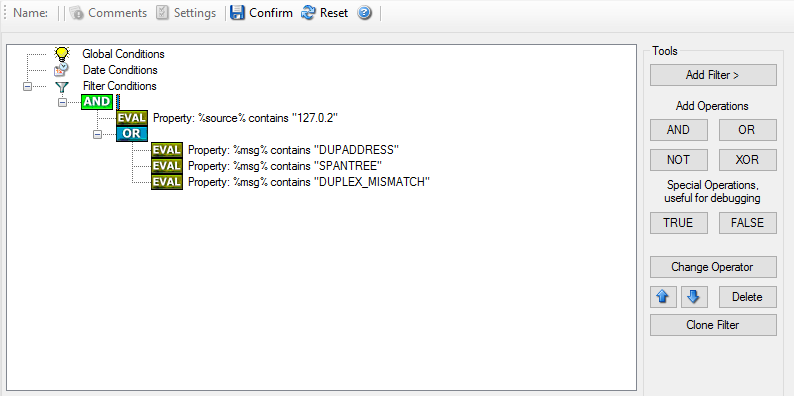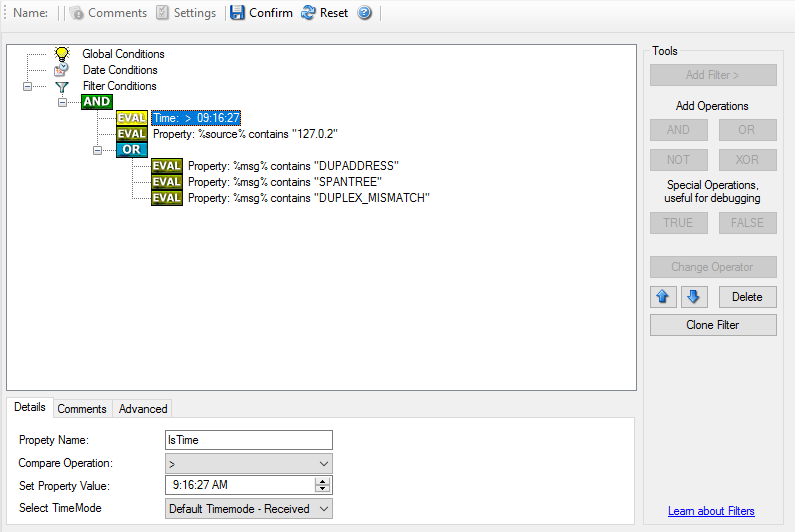# Complex Filter Conditions¶

The rule engine uses complex filter conditions.

Powerful boolean operations can be used to build filters as complex as needed. A boolean expression tree is graphically created. The configuration program is modelled after Micorosoft Network Monitor. So thankfully, many administrators are already used to this type of Interface. If you are not familiar with it, however, it looks a bit confusing at first. In this chapter, we are provinding some samples of how boolean expressions can be brought into the tree.

## Example 1¶

In this example, the message text itself shall be checked. If it contains at least one of three given strings, the filter should become true. If none of the string is found, the boolean expression tree evaluates to false, which means the associated action(s) will not be executed.

In pseudo-code, the filter could be written like this:

`If (msg  = "DUPADDRES") OR (msg = "SPANTREE") OR (msg = "DUPLEX_MISMATCH) then`

`execute action(s)`

`end if`

Please note: in the example, we have abbreviated “message” to just “msg”. Also note that for brevity reasons we use the equals (“=”) comparison operator, nicht the contains. The difference between the equals and the contains operator is that with “contains”, the string must just be part of the message.

In the filter dialog, this pseudo code looks as follows:Figure 1 - Example 1

## Example 2¶

Example 2 is very similar to example 1. Again, the message content is to be checked for three string. This time, all of these strings must be present in order for the boolean tree to evaluate to false.

The pseudo code would be as follows (under the same conditions outlined in example 1 above):

`If (msg  = "DUPADDRES") AND (msg = "SPANTREE") AND (msg = "DUPLEX_MISMATCH) then`

`execute action(s)`

`end if`

In the filter dialog, this pseudo code looks as follows:Figure 2 - Example 2

## Example 3¶

This example is a bit more complex version of example 1. Again, the same message text filtering is done, that is if any one of the provided substrings is present, the filter eventually evaluates to true. To do so, the source system must also contain the string “192.0.2”, which can be used to filter on a device from a specifc subnet.

An example like this can be used for a rule where the administrator of a specific subnet should be emailed when one of the strings indicate a specific event.

The pseudo code would be as follows (under the same conditions outlined in example 1 above):

`If ((sourceSys = "192.0.2") And`
`((msg  = "DUPADDRES") OR (msg = "SPANTREE")`
`OR (msg = "DUPLEX_MISMATCH))) then`

`execute action(s)`

`end if`

In the filter dialog, this pseudo code looks as follows:Figure 3 - Example 3

As a side note, you may want to use a range check instead of a simple include for the source system. With a range string check, you can specify that the string must be within a specified column range, in this case obviously at the beginning of the source system IP address.

## Real-World Examples¶

To see some real-world examples of where boolean conditions inside filtering are used, please visit these web links:

## Example 4¶

In this example, the report is to be filtered in such a way that it shows information only in the case, if the time is greater then certain time with certain event source and one of two event ID’s.

In pseudo-code, the filter could be written like this:

`If (DeviceReportedTime is greater than {9:16:27} AND EventSource is equal to` `{Print} AND [EventID is equal to {10} OR EventID is equal to {18}]]`

In the filter dialog, this pseudo code looks as follows: